# Data Analysis Startup

In this assignment you're going to get an introduction to very basic data manipulation using data from a current meter mooring.
To work with this file, rather than reading the html version, go to theTable of Contents and right-click on 'mlx version' to save it.
You'll need a working directory for the course. Make such a directory and put it on your Matlab search path. This is done by putting a line line 'addpath /path/to/course/files' in your startup.m file and then runnng 'startup' at Matlab's command prompt. Then put the above mlx file into that directory.
Some of this may be review, but we will establish some data organization conventions, learn some key Matlab capabilities, and be introduced to some useful functions in jLab. Along the way we'll get a preview of techniques we will study more later this week.
You'll be looking at data from a current meter mooring from the central Labrador Sea, between Greenland and Canada, known as the ‘Bravo’ mooring.
Note you can change the text size in this window using ⌘-plus or ⌘-minus.

# Topics Covered

The topics covered in this assignment are
1. Working with Matlab Live Scripts
2. Organizing data in structures
3. Simplifing the use of structures with jLab's make, use, and matsave
4. Using Matlab's datenum for dates, and jLab's yearfrac
5. Sample interval statistics with jLab's sampletimes
6. Simple smoothing with jLab's vfilt
7. Changing line styles with jLab's linestyle
8. Plotting stick vectors with jLab's stickvect
9. Plotting hodographs with jLab's hodograph
10. Plotting progressive vector diagrams with jLab's provec

# Matlab Live Scripts

For starters, you're going to be working with a Matlab Live Script. This will be a convenient way for you to organize your notes during this class and possibly in real life, too.
Evaluate the following code. The dataset bravo94 is included with jLab and should load if you have your path set correctly.
clear
bravo94
bravo94 = struct with fields:
description: 'Bravo mooring 1994-1995'
creator: 'Created by the JBRAVO script by J.M. Lilly'
timestamp: '28-Apr-2017 11:20:58'
lat: 56.7533
lon: -52.4550
rcm: [1×1 struct]
cat: [1×1 struct]
The Live Script format lets you step through a series of Matlab commands in an easy way. Instead of actually typing this on the command line, just right-click within the code block and choose ‘Run Section’. Note the output appears underneath the command. Alternatively, click to put the cursor in the code block, and then use the key command, which is ⌘-Return on a Mac. You can also click ‘Run Section’ in the menu bar. Or, if the cursor is in the section you will see a blue bar to the left that you can also click on.
In the upper right corner of the script window are two icons. Click on them to see how the output display changes. The left (or upper) one, which displays the output inline, will probably be better.
If you click on the 'Live Editor' tab in the Matlab window, you'll see a bunch of tools for working with Live Scripts. For example, the big green 'Run' arrow runs the whole script. To start over, right click and choose ‘Clear All Output.’

# Structures

bravo94 is a type of Matlab array known as a structure. The elements, or fields, of a structure can be any type of variable: character arrays, numerical arrays, or even other structures.
Each time you see a block of code below, run the section as above. If you want to start over, you can right click and choose ‘Clear All Output.’ This all happening in your workspace, by the way, so you can feel free to type in addtional commands on the command line.
bravo94.description
ans = 'Bravo mooring 1994-1995'
bravo94.lat
ans = 56.7533
bravo94.cat
ans = struct with fields:
description: 'Bravo Seacats 1994-1995'
depths: [260 510 1010 1510 2010 2512]
num: [9126×1 double]
s: [9126×6 double]
th: [9126×6 double]
sigth: [9126×6 double]
p: [9126×6 double]
bravo94.cat.depths
ans = 1×6
260 510 1010 1510 2010 2512
Based on examining these fields, do you understand the basic idea of a structure?
Adding a new field to a structure is easy.
bravo94.comment = 'Adding a new field';
bravo94.comment
ans = 'Adding a new field'
You can also manipulate fields through rmfield, setfield, and getfield, though I rarely use these.

# UsingUse

Next we'll examine a shorthand for structures in jLab.
Often one ends up with the same physical variables, e.g. temperature and velocity, in different datasets.
It becomes tedious to type things like plot(complicated_variable_name_temperature).
My solution is to let the variables have simple names, and let these be the fields of a structure, which can be mapped into memory.
whos
Name Size Bytes Class Attributes

ans 1x18 36 char
bravo94 1x1 3654894 struct
use bravo94.cat
whos
Name Size Bytes Class Attributes

ans 1x18 36 char
bravo94 1x1 3654894 struct
depths 1x6 48 double
description 1x23 46 char
num 9126x1 73008 double
p 9126x6 438048 double
s 9126x6 438048 double
sigth 9126x6 438048 double
th 9126x6 438048 double
See how after the command use bravo94.cat,all the fields of bravo94.cat are now variables in memory?

# UseandMake

Now we can use one plot command, and just swap out the variables.
plot(th),axis tight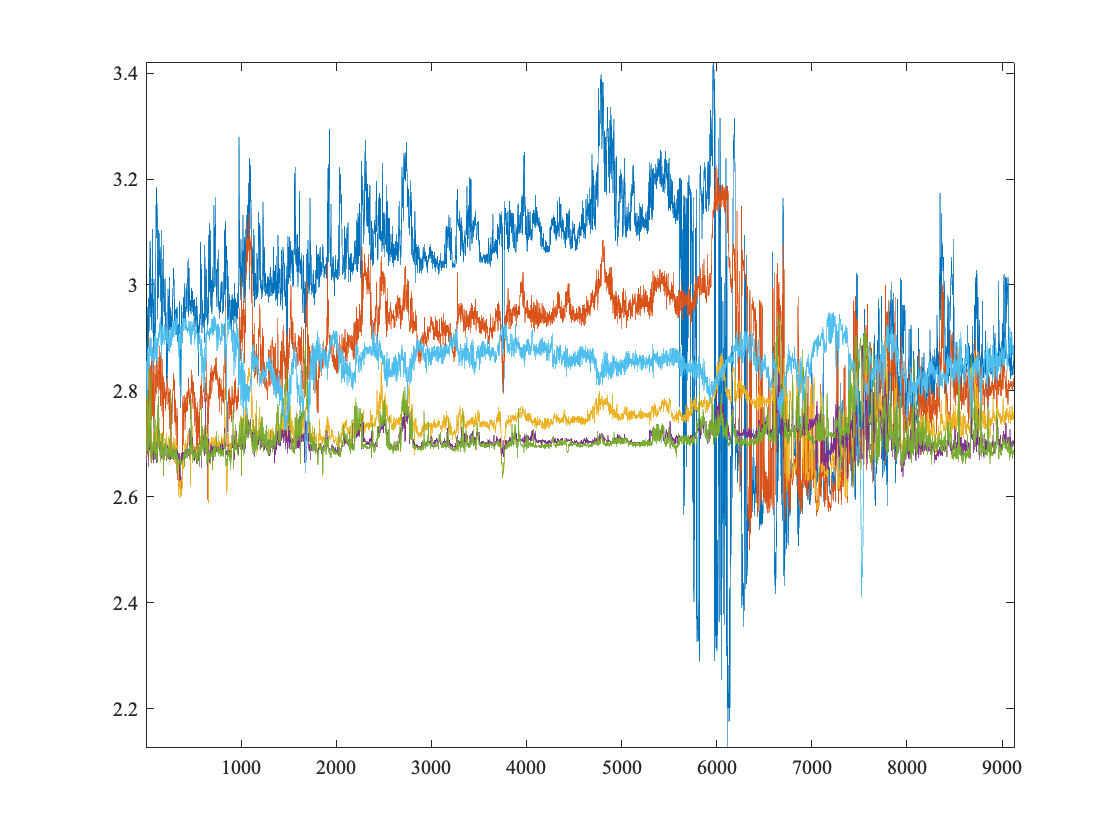use bravo94.rcm
plot(th),axis tight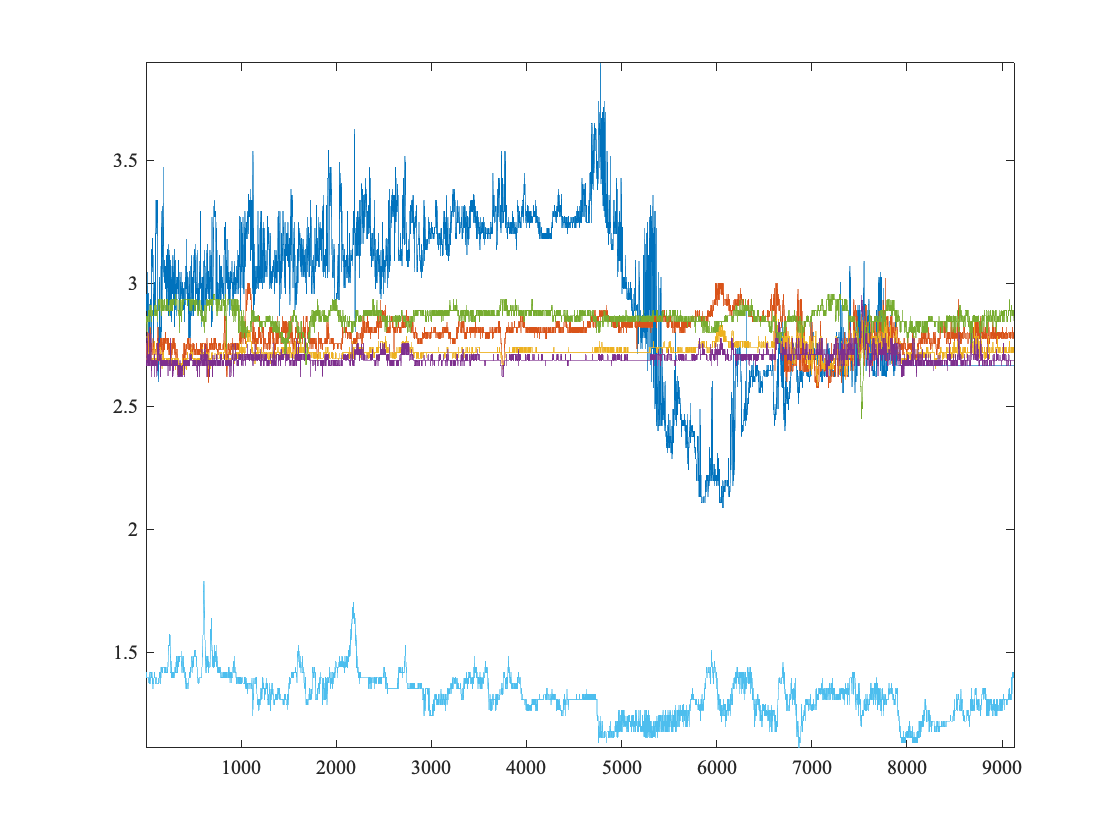I find this to be an easy way to make common plots without having to change the names of the plotted variables all the time.
The first command plots the potential temperatures, θ, associated with the SeaCat instruments, and the second plots the potential temperatures associated with the current meters.
This is all just a pitch for organizing your data into structures.
There is also a shorthand for creating a new structure in jLab.
x=1:10;
string='Just checking';
make example string x
example
example = struct with fields:
string: 'Just checking'
x: [1 2 3 4 5 6 7 8 9 10]
So make name var1 var2 ... creates a structure called name with var1, var2, etc. as fields. matsave does the same thing as make, but also saves the resulting structure as a .mat file.

# Date Conventions

Dates in Matlab are typically represented using datenum. datenum returns a kind of Julian day, with day 1 corresponding to January 1 in the fictional year 0000. My convention is that the datenum variable is always called ‘num’.
A datenum can be converted back to a string using datestr:
datestr(num(1))
ans = '28-May-1994 02:00:00'
datestr(num(end))
ans = '12-Jun-1995 07:00:00'
It can be more convenient for plotting purposes to express time in ‘Year Dot Fraction’ format, using jLab's yearfrac function.
yearfrac(num(1))
ans = 1.9944e+03
floor(yearfrac(num(1))) %Year portion
ans = 1994
yearfrac(num(1))-floor(yearfrac(num(1))) %fraction portion
ans = 0.4030
Let's redo the above plot but with year.fraction as the time base.
use bravo94.cat
plot(yearfrac(num),th),axis tight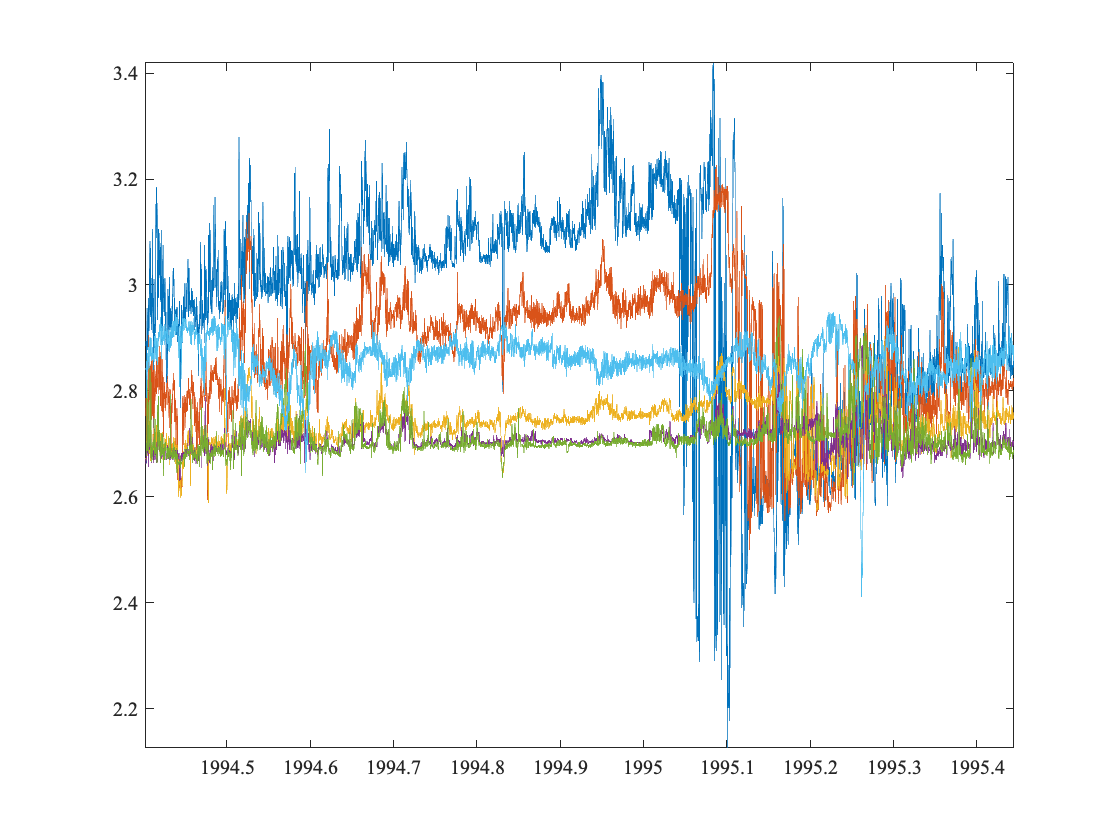That's nicer, isn't it?
[yf,mf]=yearfrac(num); will output the 'Month Dot Fraction' in its second argument. I find the year.fraction and month.fraction to be very handy for dealing with dates.
Let's say we want to use month and not year as our time base for a plot. The dataset spans 1994 and 1995, so we can do this by adding 12 to any months occuring during 1995.
[yf,mf]=yearfrac(num);
mf=mf+12*(yf>=1995);
plot(mf,th),axis tight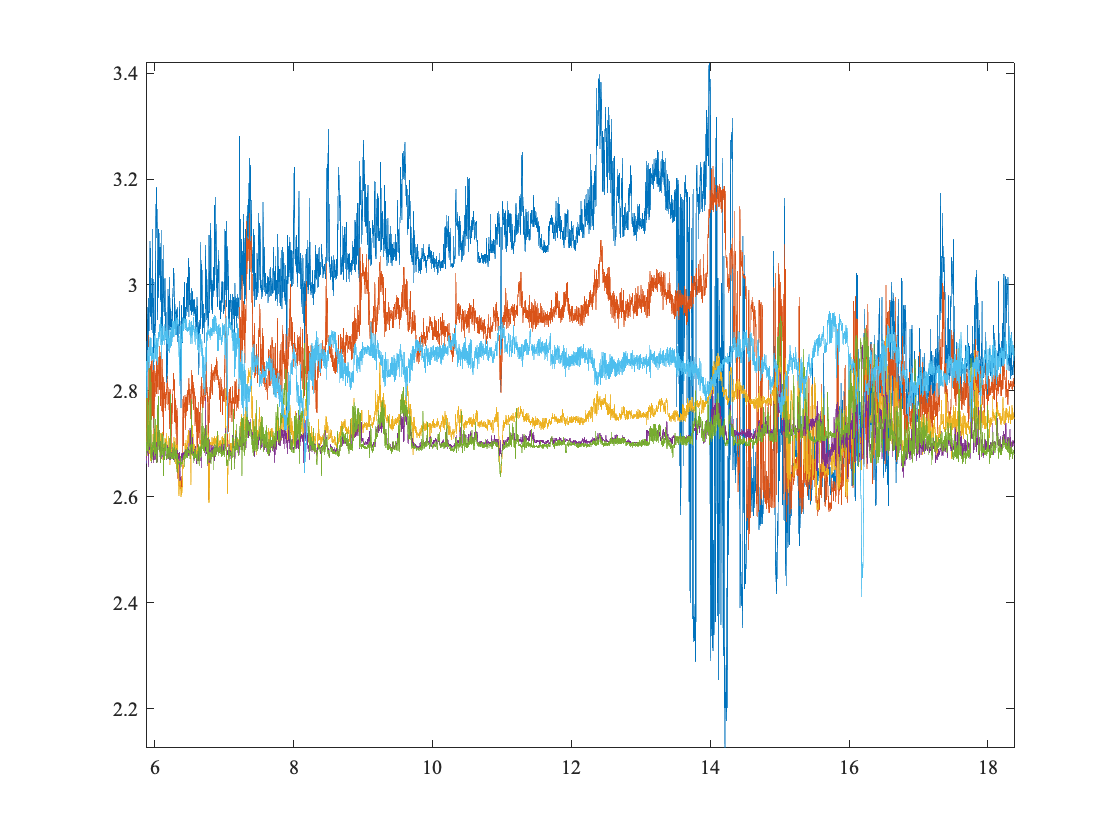In the above, "(yf>=1995)" is a boolean (true/false) operator that returns true (or one) when the year is greater than or equal to 1995, and zero otherwise. Boolean operators are super useful, as in this example.
For working with dates, Matlab also has a fancy new array type called datetime, though I haven't used it.

# The Sampling Inteval

One of the first things to do with a new dataset is to examine the sampling intervals, that is, the time period between data points. The duration of the sampling interval tells you a lot about what types of physical processes are expected to be resolved. As we shall see, it also determines the upper limit of what frequencies can be observed.
If the sampling interval is uniform, you can find it with
num(2)-num(1)
ans = 0.0417
which is recognized as 1/24 of a day, or one hour.
For mooring data, the sampling interval is generally uniform. But for many other types of data, the sampling interval is not uniform, and in those cases we need to do additional processing.
A convenient jLab function for examining the sampling interval is called sampletimes.
use bravo94.rcm
[dt,sigdt,meddt,maxdt]=sampletimes(num);
dt,sigdt,meddt,maxdt
dt = 0.0417
sigdt = 5.4876e-11
meddt = 0.0417
maxdt = 0.0417
Here dt is the mean sampling interval, sigdt (for sigma-dt) is the standard deviation of sampling intervals or times between adjacent points, meddt (for median-dt) is the median sampling interval, and datetime is the maximum sampling interval.
Running the above code, we see that the sigdt is essentially zero, and the median and maximum dt's are the same as the mean. Thus, we say this dataset has a uniform sampling interval or is uniformly sampled.

# Simple Plots

Next we'll look at simple plots of the velocity. I find it useful to represent horizontal velocity as a complex-valued number u+iv I call this variable cv=u+iv, for ‘complex velocity’.
cv=cv(:,2); %Use only the second column
uvplot(yearfrac(num),cv),axis tight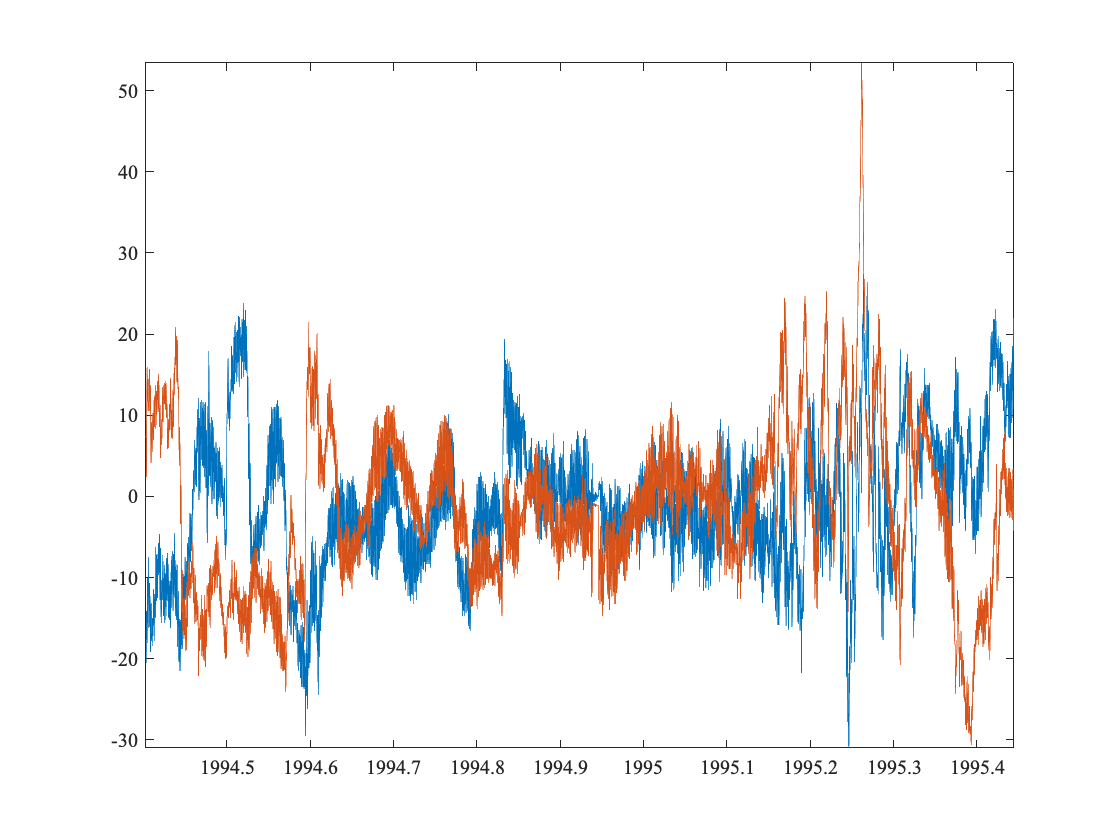uvplot is a jLab function for plotting lots both the real (eastward) and imaginary (northward) parts of the velocity.
We notice three things right away. First, the timeseries have a ‘fuzzy’ appearance, and second, there appear to be some sharp transitions where one or both velocity components change suddenly. This is particularly evident in the first half of the record. Finally, we see that there is some temporal variability, with a quiescent central time period and a more energetic final third of the record.
Next we will apply a simple filter. Note we're using clf each time we make a new plot as the Live Script essentially recycles the same figure window. Otherwise we would see our whole history of plots overlaid on each other.
clf, uvplot(yearfrac(num),vfilt(cv,24)),axis tight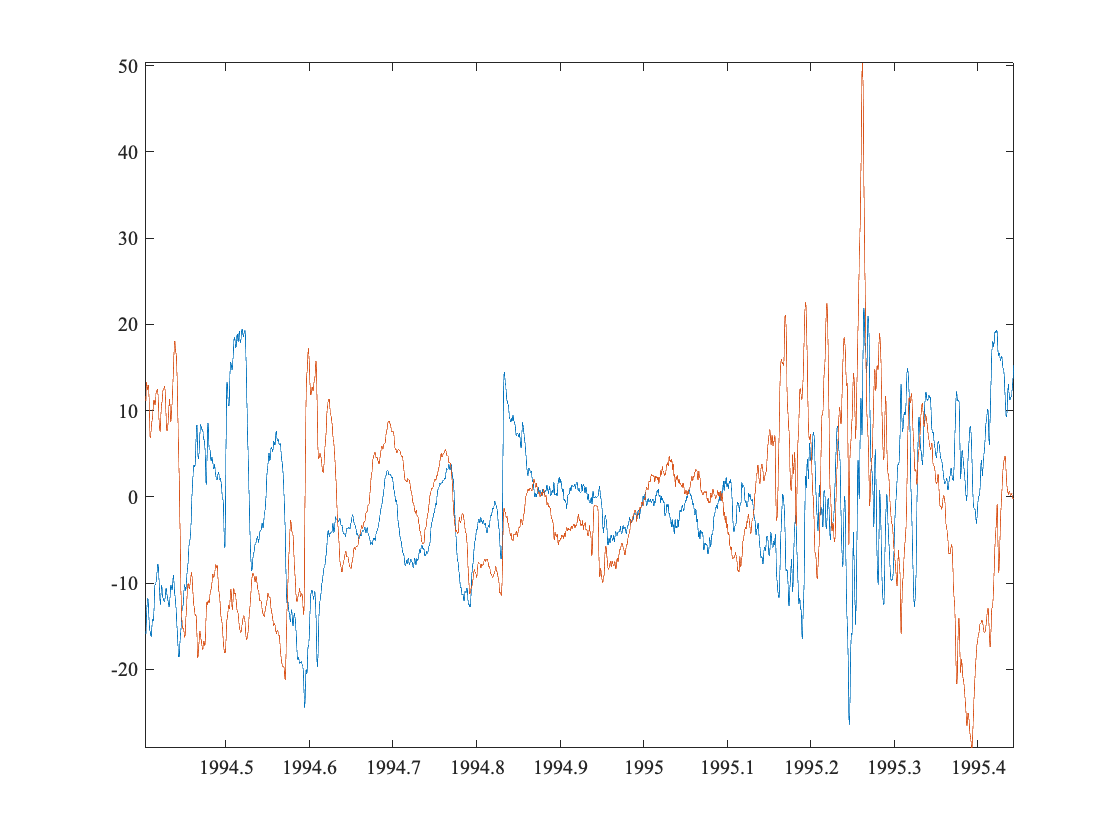The command vfilt(x,N) applies an N-point Hanning filter to the column vector x. With hourly sampling, this 24-point filter is sufficient to remove most semidiurnal tidal variability, as well as inertial variabilty which at this latitude has about a 14 hour period.
It is clear that the ‘fuzziness’ has gone away, making the sharp transitions stand out more. These transitions will be seen to be due to coherent eddies.
Let's plot the smoothed and raw data on top of each other.
clf, uvplot(yearfrac(num),cv), hold on
uvplot(yearfrac(num),vfilt(cv,24)), axis tight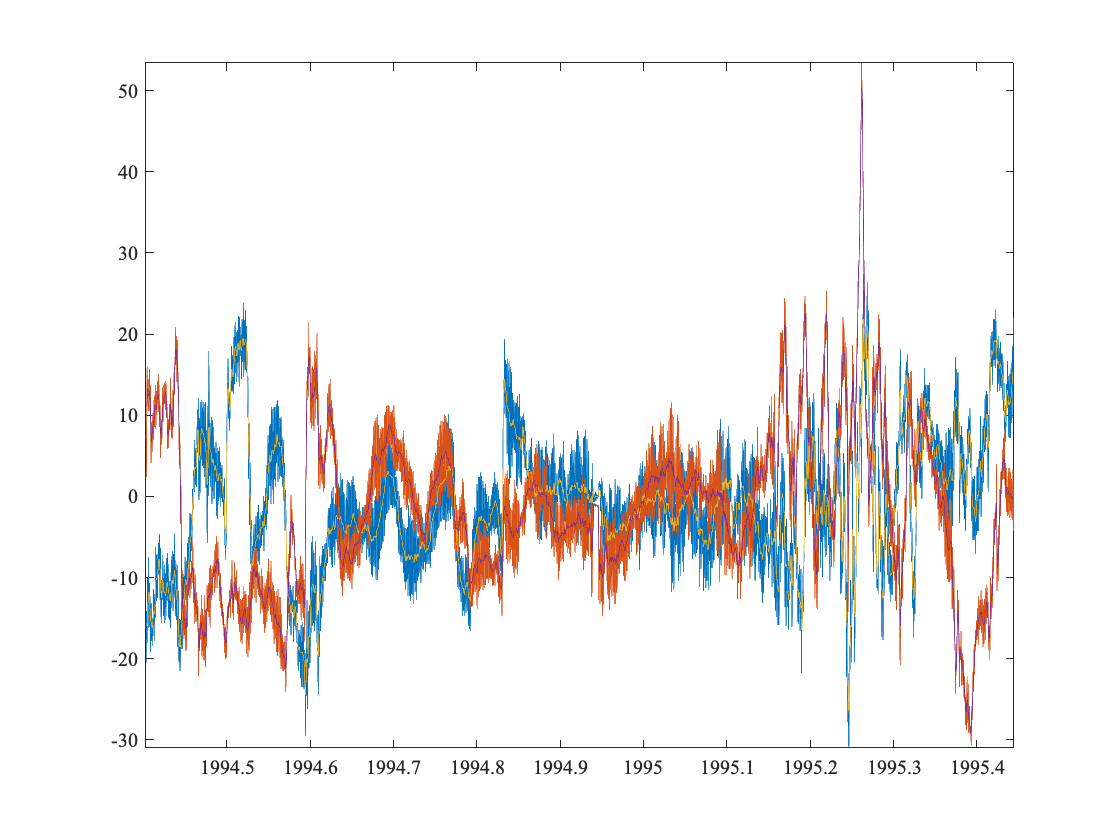Go ahead and zoom in. You can clearly see the oscillations of the unfiltered data around the filtered data. Later, when we get to spectra, we will show that these are tidal and inertial oscillations.
It's a little hard to see the different lines, so now we'll change the linestyles.

# Changing Line Styles

In jLab there is an easy way to set line styles and colors
clf, uvplot(yearfrac(num),cv), hold on
uvplot(yearfrac(num), vfilt(cv,24)), axis tight
linestyle T U 2k 2G